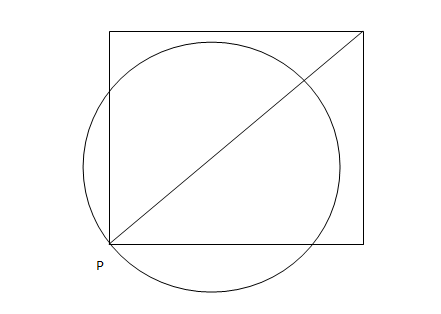# CAT 2006

Instructions

A punching machine is used to punch a circular hole of diameter two Units from a square sheet of aluminium of width 2 units, as shown below. The hole is punched such that the circular hole touches one corner P of the square sheet and the diameter of the hole originating at P is in line with a diagonal of the square:Question 1

# The proportion of the sheet area that remains after punching is:Question 2

# Find the area of the part of the circle (round punch) falling outside the square sheet.Instructions

An airline has a certain free luggage allowance and charges for excess luggage at a fixed rate per kg. Two passengers, Raja and Praja have 60 kg of luggage between them, and are charged Rs 1200 and Rs 2400 respectively for excess luggage. Had the entire luggage belonged to one of them, the excess luggage charge would have been Rs 5400.

Question 3

# What is the weight of Praja’s luggage?Question 4

# What is the free luggage allowance?Instructions

For the following questions answer them individually

Question 5

# If x = -0.5, then which of the following has the smallest value?Question 6

# Which among $$2^{1/2}, 3^{1/3}, 4^{1/4}, 6^{1/6}$$, and $$12^{1/12}$$ is the largest?Question 7

# If a/b = 1/3, b/c = 2, c/d = 1/2 , d/e = 3 and e/f = 1/4, then what is the value of abc/def ?Question 8

# The length, breadth and height of a room are in the ratio 3:2:1. If the breadth and height are halved while the length is doubled, then the total area of the four walls of the room willQuestion 9

# Consider a sequence where the $$n^{th}$$ term, $$t_n = n/(n+2), n =1, 2, ....$$ The value of $$t_3 * t_4 * t_5 * …..* t_{53}$$ equals.Question 10

# A group of 630 children is arranged in rows for a group photograph session. Each row contains three fewer children than the row in front of it. What number of rows is not possible?OR